### Home > CALC > Chapter 11 > Lesson 11.1.1 > Problem11-10

11-10.
1. If vector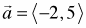,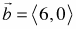, and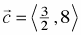, find: Homework Help ✎

1.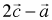2.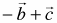3.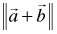4.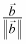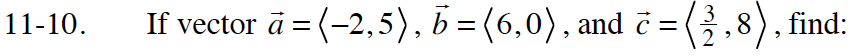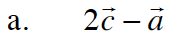$2\big<\frac{3}{2},8\big>-\big<-2,5\big>$

$\big<3,16\big>-\big<-2,5\big>$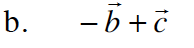$-\big<6,0\big>+\Big<\frac{3}{2},8\Big>$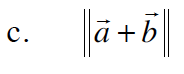$\text{Express }\vec{a}+\vec{b}\text{ as a single vector.}$

The double bars indicate "the length of". Use the Pythagorean Theorem to calculate this length.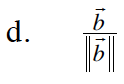$||\vec{b}||=6$